Neural Network - DigitClassifier                                 Home : www.sharetechnote.com

Digit Classifier

In this tutorial, I will apply the forwardnet to solve more like a real life problem.. but I have simplified some parts (like training data) just to show you the whole process in this short tutorial. So this example may not be applicable for the data set with wide variation but you would get a sense of overall process of applying a neural network from data preparation to solution finding.

Hidden Layer Size : 30 Neurons and 1 Output

Step 1 : Preparation of Labeled Image

To apply a neural net for digit classification (a type of image classification application), first you need to prepare a set of labeled image. This can be a good example of various data preparation that I mentioned in what they do page. There can be various way to prepare the labeled data for the training, but the way I take is as follows.

i) First, I created 10 folders with the folder name matching the label for the images that are stored in the folder. i.e, I created 10 folders with name 0,1,2,3,4,5,6,7,8,9 as shown below.

ii) Then, I put the images with numbers written on it.

NOTE 1 : For simplicity, I have created all the image files with the same size. In real situation, you may get all of these images with different size.

NOTE 2 : To reduce the size of input layer and hidden layers I have written numbers in a very small image (just 10 x 10 pixels).

NOTE 3 : As another measure for simplification of data preparation, I put only single image for each label. It mean that this network would not work very well in real life situation with a lot of variations of image.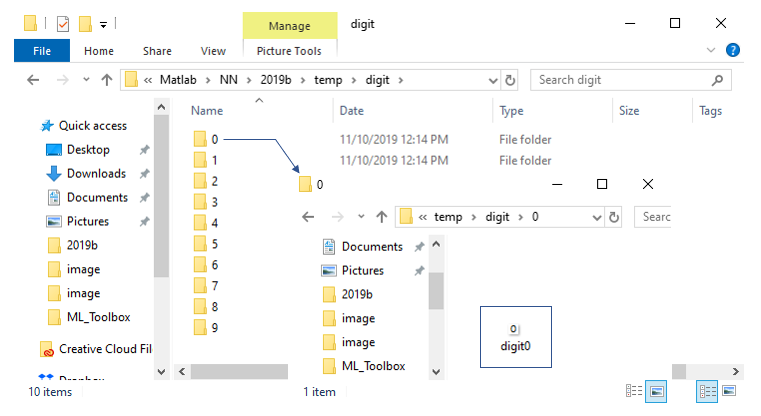Step 2 :  Read the labeled image and construct the training data

In this step, I am going to read all the files from the folders and convert the 2D images into 1D array(vector) to transfer it to the input layer of the network.

dv = [];

for i = 0:9

fn = sprintf('%s\\temp\\digit\\%d\\digit%d.png',pwd,i,i);

imgBW = rgb2gray(img);

subplot(2,5,i+1);

imshow(imgBW,'InitialMagnification','fit');

dv = [dv reshape(imgBW',[],1)];

end

Following is to read a file from a folder and save it to a variable img. At this point, the img would contain a matrix with the size of 100 x 100 x 3. 100 x 100 is image size and 3 is number of RGB Color layer for the image.

fn = sprintf('%s\\temp\\digit\\%d\\digit%d.png',pwd,i,i);

For simplicity, I will design a network that can take in single layer image (i.e, gray scale color). So I need to convert the 3 layer color image into a single layer grayscale image using followig function.

imgBW = rgb2gray(img);

If I plot all the images into a single window using the following code, it is as shown below.

subplot(2,5,i+1);

imshow(imgBW,'InitialMagnification','fit');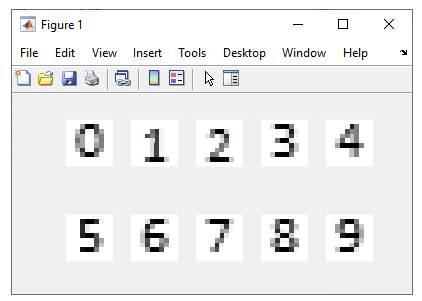Now convert a 2D image matrix into a 1D vector using reshape() function and consolidate all the image vector into a matrix named dv as show below.

dv = [dv reshape(imgBW',[],1)];

Now I added a line which is only for Matlab requirement, nothing to do with neural network algorith. I just convert the matrix dv (which is all integer element) to double type.

dv = double(dv);

Now I define the output vector that will be used in train stage later. In this example, I am labeling the image of number 0 as the number 0.0, the image of number 1 as 0.1 an so on.

dvc = [0 1 2 3 4 5 6 7 8 9]/10.0;

Step 3 :  Construct a Neural Net

Now I create a network with following structure. This has 100 inputs that are connected to 30 neurons and it has one output which produce the number 0.0, 0.1, 0.2 ...., 0.9.

net = feedforwardnet(30);

As you see here, the number 30 indicates the number of neurons in the hidden layer. This function does not specify the number of output cell. Then how the number of output cell is determined ? Not in this step. It will be automatically determined by the structure of traiing output vector at training stage which I will show you later.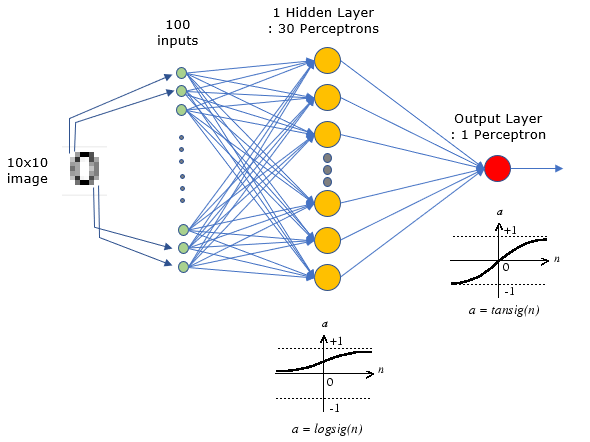Step 4 :  Configure the detailed network Parameter

Now I set some detailed parameter (like loss (error) calculation algorithm, weight/bias update algorithm and activation function type for each layer.

net.performFcn = 'mse';   % loss/error calculation

net.trainFcn = 'trainlm';    % weight/bias update algorithm (back propagation algorithm)

net.divideFcn = 'dividetrain';

net.layers{1}.transferFcn = 'logsig';  % activation function type for Hidden layer

net.layers{2}.transferFcn = 'tansig'; % activation function type for output layer

Step 5 :  Train the network

Now let me set some options for training options as follows.

net.trainParam.epochs =1000;

net.trainParam.goal = 10^-1;

net.trainParam.max_fail = 1;

Now perform the training for the network as follows.

net = train(net,dv,dvc);

the variable dv is the input vectors made up of 10 image files as shown below and dvc is a vector that indicates the label (the expected output) as indicated below.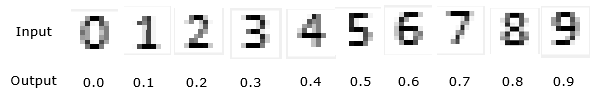Training went as follows.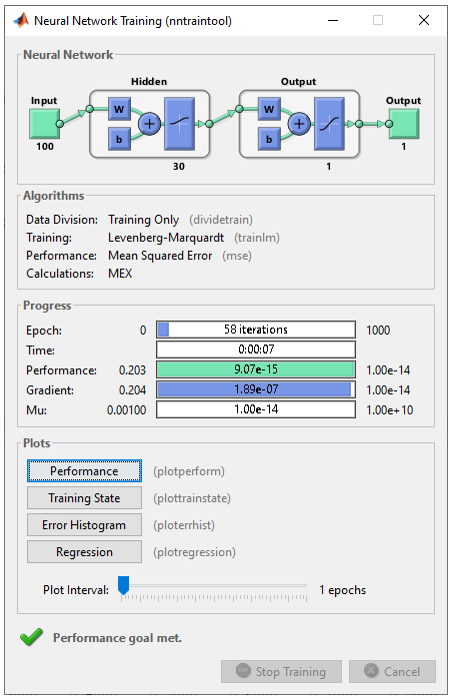Following plots shows how the training proceeded. I would not explain any details on these plots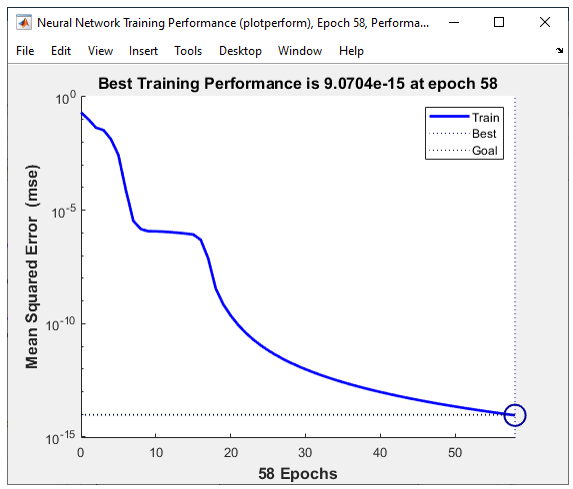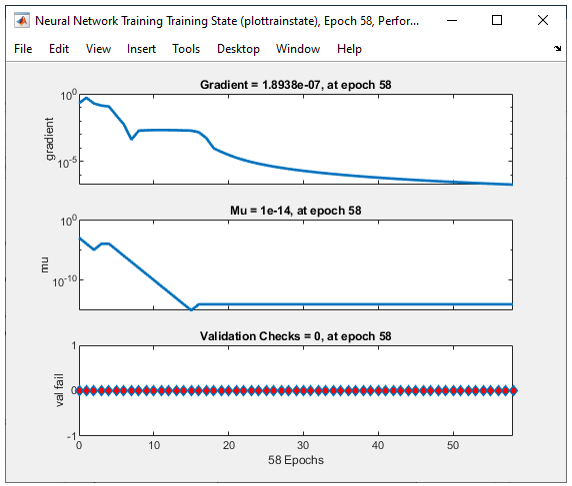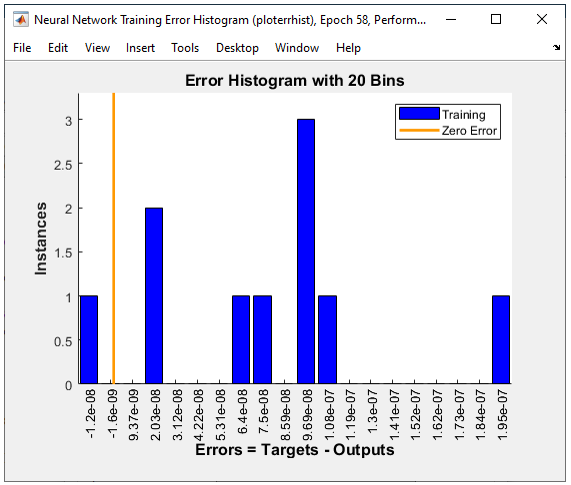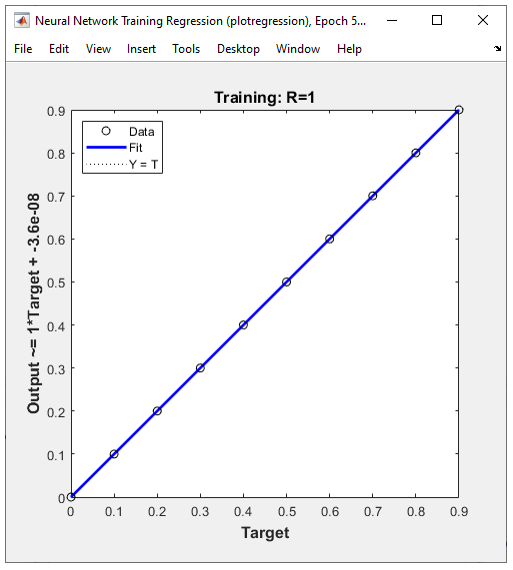% read file and preprocess the file dv = []; for i = 0:9     fn = sprintf('%s\\temp\\digit\\%d\\digit%d.png',pwd,i,i);     img=imread(fn);     imgBW = rgb2gray(img);     subplot(2,5,i+1);     imshow(imgBW,'InitialMagnification','fit');     dv = [dv reshape(imgBW',[],1)]; end   set(gcf,'Position',[100 100 400 200]);   dv = double(dv); dvc = [0 1 2 3 4 5 6 7 8 9]/10.0;   % create the network net = feedforwardnet(30);     % configure the network parameters net.performFcn = 'mse'; net.trainFcn = 'trainlm'; net.divideFcn = 'dividetrain'; net.layers{1}.transferFcn = 'logsig'; net.layers{2}.transferFcn = 'tansig';   % configure training parameters net.trainParam.epochs =1000; net.trainParam.goal = 10^-14; net.trainParam.min_grad = 10^-14; net.trainParam.max_fail = 1; %net.trainParam.ValidationChecks = 100;   % perform training net = train(net,dv,dvc);   % verify with test data y = net(dv)

Hidden Layer Size : 30 Neurons and 10 output

This tutorial is almost same as the previous one. The only difference is the output type. In previous example, each image (digit) is labeled as a single number, but in this example each image (digit) is labeled as a vector as in step 2.

Step 1 : Preparation of Labeled Image

Step 1 is almost identical to the step 1 in previous example. So I would not explain again in this tutorial.  See the step 1 of the previous section for the details of data preparation.

Step 2 :  Read the labeled image and construct the training data

In this step, I am going to read all the files from the folders and convert the 2D images into 1D array(vector) to transfer it to the input layer of the network.

dv = [];

for i = 0:9

fn = sprintf('%s\\temp\\digit\\%d\\digit%d.png',pwd,i,i);

imgBW = rgb2gray(img);

subplot(2,5,i+1);

imshow(imgBW,'InitialMagnification','fit');

dv = [dv reshape(imgBW',[],1)];

end

Following is to read a file from a folder and save it to a variable img. At this point, the img would contain a matrix with the size of 100 x 100 x 3. 100 x 100 is image size and 3 is number of RGB Color layer for the image.

fn = sprintf('%s\\temp\\digit\\%d\\digit%d.png',pwd,i,i);

For simplicity, I will design a network that can take in single layer image (i.e, gray scale color). So I need to convert the 3 layer color image into a single layer grayscale image using followig function.

imgBW = rgb2gray(img);

If I plot all the images into a single window using the following code, it is as shown below.

subplot(2,5,i+1);

imshow(imgBW,'InitialMagnification','fit');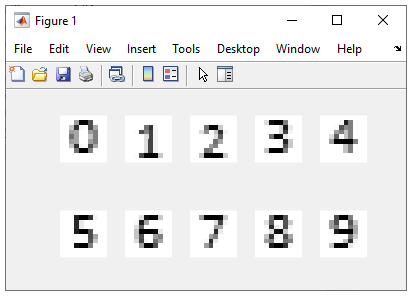Now convert a 2D image matrix into a 1D vector using reshape() function and consolidate all the image vector into a matrix named dv as show below.

dv = [dv reshape(imgBW',[],1)];

Now I added a line which is only for Matlab requirement, nothing to do with neural network algorith. I just convert the matrix dv (which is all integer element) to double type.

dv = double(dv);

Now I define the output vector that will be used in train stage later. In this example, I am labeling the image of number 0 as the vector [1 0 0 0 0 0 0 0 0 0] , the image of number 1 as vector [0 1 0 0 0 0 0 0 0 0] an so on.

dvc = [1 0 0 0 0 0 0 0 0 0;

0 1 0 0 0 0 0 0 0 0;

0 0 1 0 0 0 0 0 0 0;

0 0 0 1 0 0 0 0 0 0;

0 0 0 0 1 0 0 0 0 0;

0 0 0 0 0 1 0 0 0 0;

0 0 0 0 0 0 1 0 0 0;

0 0 0 0 0 0 0 1 0 0;

0 0 0 0 0 0 0 0 1 0;

0 0 0 0 0 0 0 0 0 1];

Step 3 :  Construct a Neural Net

Now I create a network with following structure. This has 100 inputs that are connected to 30 neurons and it has one output which produce the vector [1 0 0 0 0 0 0 0 0 0], [0 1 0 0 0 0 0 0 0 0] and so on.

net = feedforwardnet(30);

As you see here, the number 30 indicates the number of neurons in the hidden layer. This function does not specify the number of output cell. Then how the number of output cell is determined ? Not in this step. It will be automatically determined by the structure of traiing output vector at training stage which I will show you later.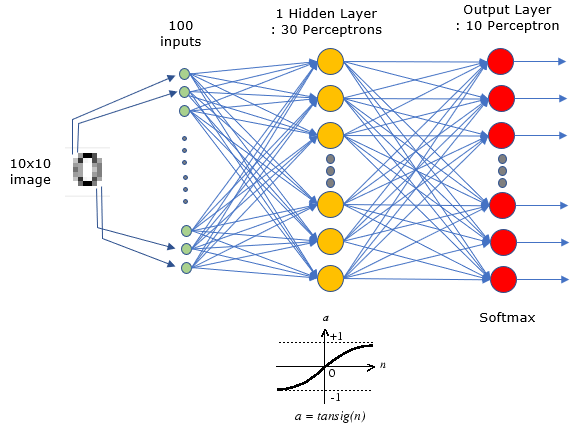Step 4 :  Configure the detailed network Parameter

Now I set some detailed parameter (like loss (error) calculation algorithm, weight/bias update algorithm and activation function type for each layer.

net.performFcn = 'mse';   % loss/error calculation

net.trainFcn = 'trainlm';    % weight/bias update algorithm (back propagation algorithm)

net.divideFcn = 'dividetrain';

net.layers{1}.transferFcn = 'logsig';  % activation function type for Hidden layer

net.layers{2}.transferFcn = 'softmax'; % activation function type for output layer

Step 5 :  Train the network

Now let me set some options for training options as follows.

net.trainParam.epochs =1000;

net.trainParam.goal = 10^-1;

net.trainParam.max_fail = 1;

Now perform the training for the network as follows.

net = train(net,dv,dvc);

the variable dv is the input vectors made up of 10 image files as shown below and dvc is a vector that indicates the label (the expected output) as indicated below.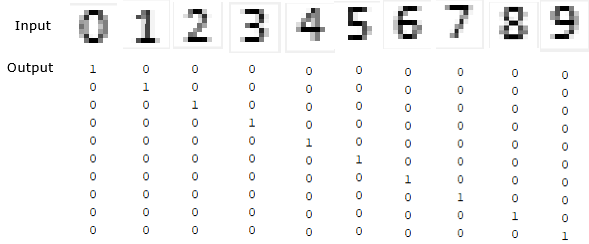Training went as follows.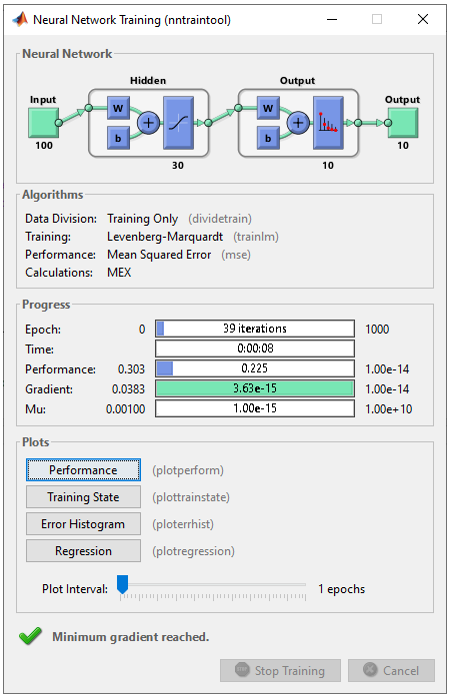Following plots shows how the training proceeded. I would not explain any details on these plots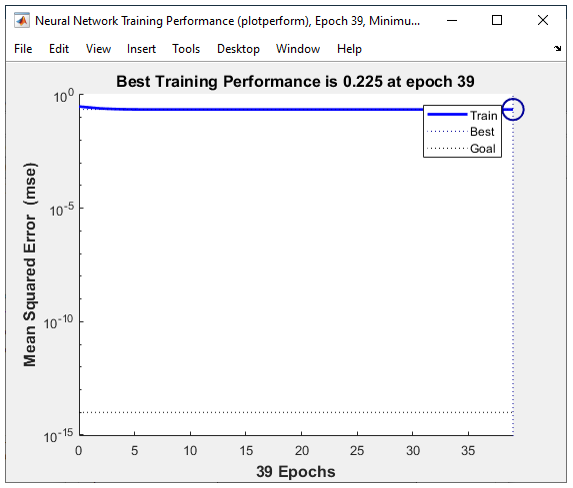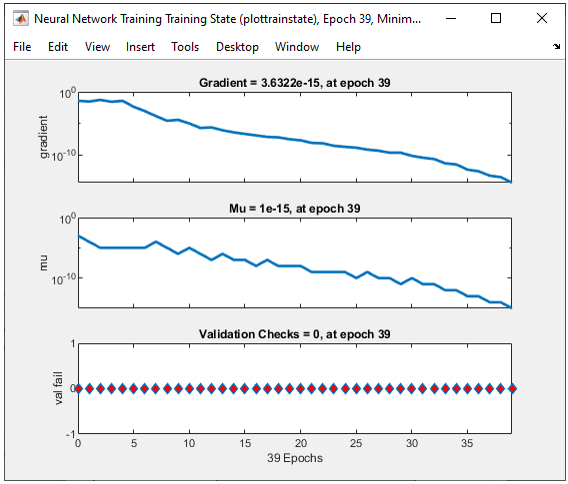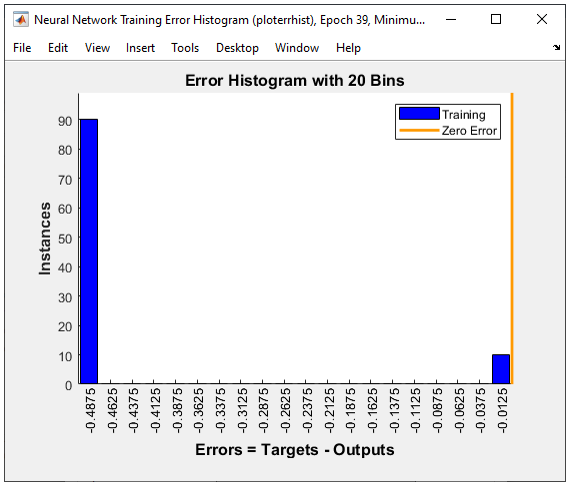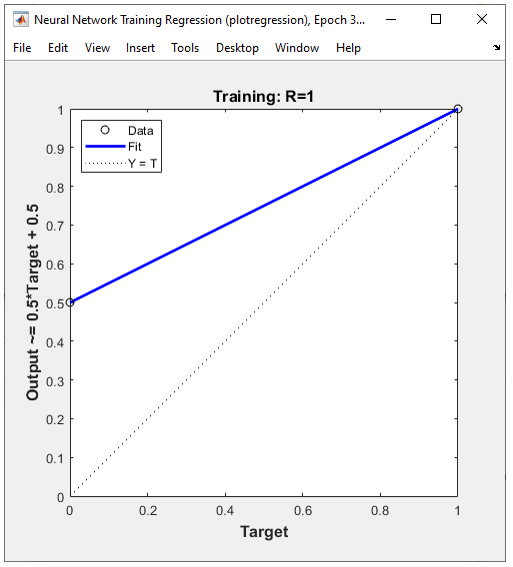dv = []; for i = 0:9     fn = sprintf('%s\\temp\\digit\\%d\\digit%d.png',pwd,i,i);     img=imread(fn);     imgBW = rgb2gray(img);     subplot(2,5,i+1);     imshow(imgBW,'InitialMagnification','fit');     dv = [dv reshape(imgBW',[],1)]; end set(gcf,'Position',[100 100 400 200]);   %dv = 1.0-double(dv)/255.0; dv = double(dv); dvc = [1 0 0 0 0 0 0 0 0 0;        0 1 0 0 0 0 0 0 0 0;        0 0 1 0 0 0 0 0 0 0;        0 0 0 1 0 0 0 0 0 0;        0 0 0 0 1 0 0 0 0 0;        0 0 0 0 0 1 0 0 0 0;        0 0 0 0 0 0 1 0 0 0;        0 0 0 0 0 0 0 1 0 0;        0 0 0 0 0 0 0 0 1 0;        0 0 0 0 0 0 0 0 0 1];     net = feedforwardnet(30);     % net = perceptron; net.performFcn = 'mse'; net.trainFcn = 'trainlm'; net.divideFcn = 'dividetrain'; net.layers{1}.transferFcn = 'tansig'; net.layers{2}.transferFcn = 'softmax';     % Perform Training   net.trainParam.epochs =1000; net.trainParam.goal = 10^-14; net.trainParam.min_grad = 10^-14; net.trainParam.max_fail = 1; %net.trainParam.mu = 5; %net.trainParam.ValidationChecks = 100;   net = train(net,dv,dvc);     y = net(dv)

Next Step :